# Chapter 3 Ratios and Rates Part 3 Day

• Slides: 39Chapter 3 Ratios and Rates (Part 3)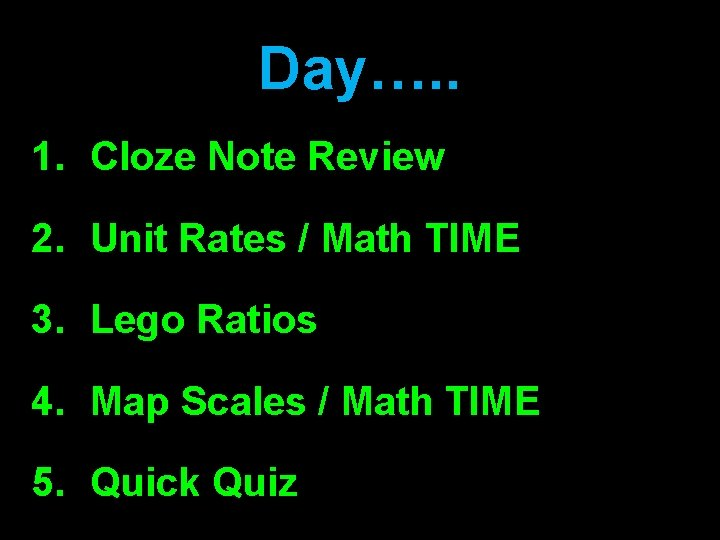Day…. . 1. Cloze Note Review 2. Unit Rates / Math TIME 3. Lego Ratios 4. Map Scales / Math TIME 5. Quick QuizDay 1Bell Work White Book Pages 5 -6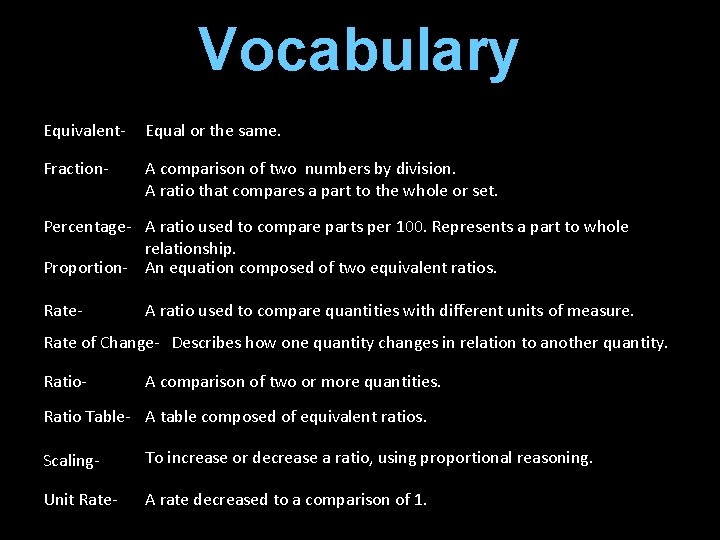Vocabulary Equivalent- Equal or the same. Fraction- A comparison of two numbers by division. A ratio that compares a part to the whole or set. Percentage- A ratio used to compare parts per 100. Represents a part to whole relationship. Proportion- An equation composed of two equivalent ratios. Rate- A ratio used to compare quantities with different units of measure. Rate of Change- Describes how one quantity changes in relation to another quantity. Ratio- A comparison of two or more quantities. Ratio Table- A table composed of equivalent ratios. Scaling- To increase or decrease a ratio, using proportional reasoning. Unit Rate- A rate decreased to a comparison of 1.I Can…. Critique the reasoning of others.Test Critique Please clear your desk of everything except for you graded test and a red ink pen.Cloze Notes We will now review the skills necessary to reason proportionally.Wrap it Up • Review • Questions • Exit TicketsDay 2Bell Work White Book Pages 19 -20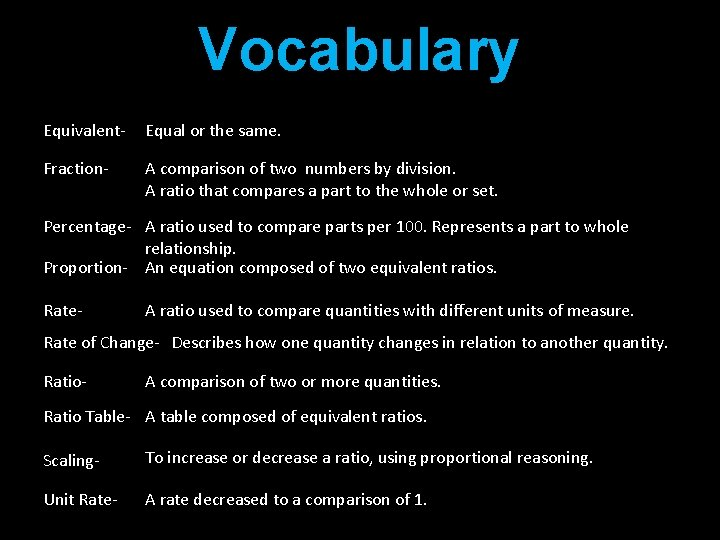Vocabulary Equivalent- Equal or the same. Fraction- A comparison of two numbers by division. A ratio that compares a part to the whole or set. Percentage- A ratio used to compare parts per 100. Represents a part to whole relationship. Proportion- An equation composed of two equivalent ratios. Rate- A ratio used to compare quantities with different units of measure. Rate of Change- Describes how one quantity changes in relation to another quantity. Ratio- A comparison of two or more quantities. Ratio Table- A table composed of equivalent ratios. Scaling- To increase or decrease a ratio, using proportional reasoning. Unit Rate- A rate decreased to a comparison of 1.Homework Check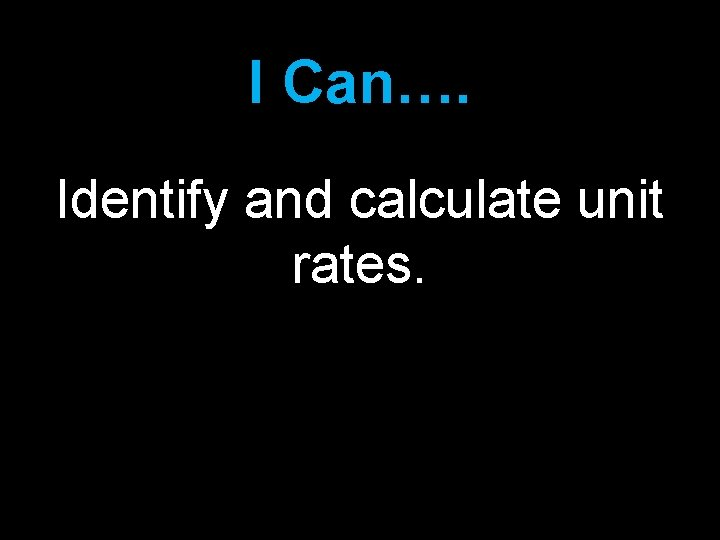I Can…. Identify and calculate unit rates.Unit Rates Essential Understanding: • A unit rate is a rate that has been reduced to a comparison of 1. Example: • A rate can be reduced by I. Using division- To use this method begin by expressing the rate in the correct order using a fraction bar. (Don’t forget your units). Then you simply divide the top quantity by the bottom quantity. Finally write the quotient as a comparison to 1. II. Using proportional reasoning- This method uses a proportion, in which the first ratio is the given rate and the equivalent ratio is set up to have a denominator of one. To determine the numerator of the equivalent ratio, simply calculate the scale factor of the denominators and use it to scale the given numerator. III. Using cross products/ One step equations. -This method uses your knowledge of cross products to create a one-step equation when you are unable to scale a proportion mentally.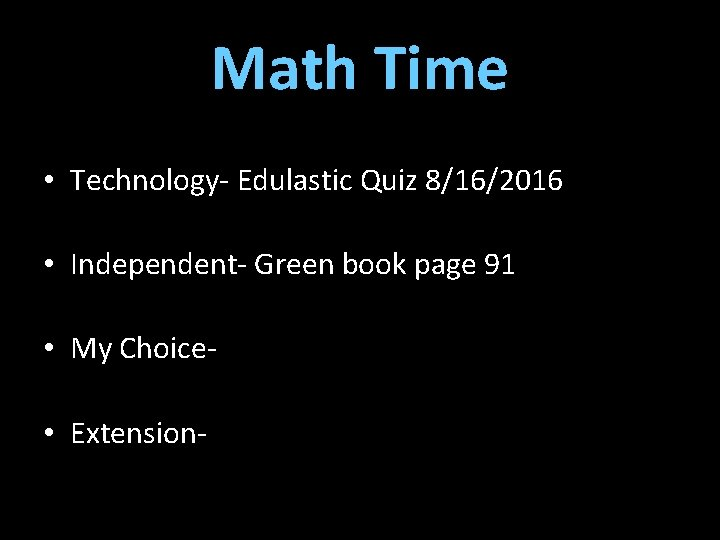Math Time • Technology- Edulastic Quiz 8/16/2016 • Independent- Green book page 91 • My Choice • Extension-Wrap it Up • Review • Questions • Exit TicketsDay 3Bell Work White Book Pages 51 -52Vocabulary Equivalent- Equal or the same. Fraction- A comparison of two numbers by division. A ratio that compares a part to the whole or set. Percentage- A ratio used to compare parts per 100. Represents a part to whole relationship. Proportion- An equation composed of two equivalent ratios. Rate- A ratio used to compare quantities with different units of measure. Rate of Change- Describes how one quantity changes in relation to another quantity. Ratio- A comparison of two or more quantities. Ratio Table- A table composed of equivalent ratios. Scaling- To increase or decrease a ratio, using proportional reasoning. Unit Rate- A rate decreased to a comparison of 1.Homework CheckI Can…. Create and scale models.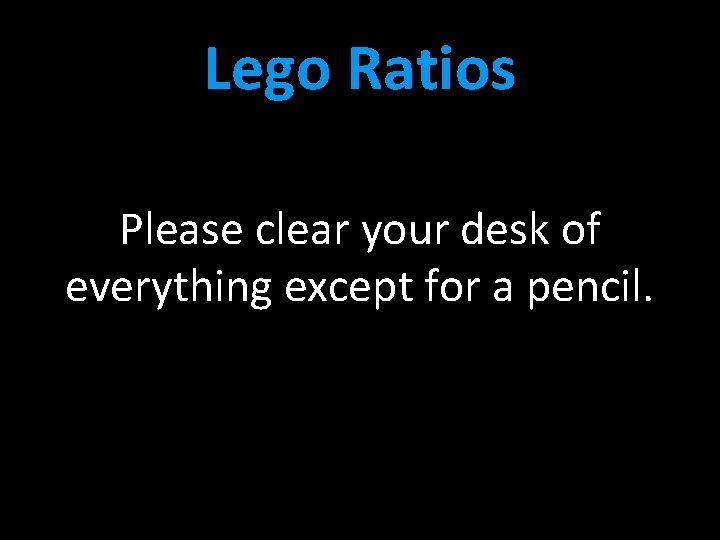Lego Ratios Please clear your desk of everything except for a pencil.Wrap it Up • Review • Questions • Exit TicketsDay 4Bell Work White Book Pages 55 -56Vocabulary Equivalent- Equal or the same. Fraction- A comparison of two numbers by division. A ratio that compares a part to the whole or set. Percentage- A ratio used to compare parts per 100. Represents a part to whole relationship. Proportion- An equation composed of two equivalent ratios. Rate- A ratio used to compare quantities with different units of measure. Rate of Change- Describes how one quantity changes in relation to another quantity. Ratio- A comparison of two or more quantities. Ratio Table- A table composed of equivalent ratios. Scaling- To increase or decrease a ratio, using proportional reasoning. Unit Rate- A rate decreased to a comparison of 1.Homework Check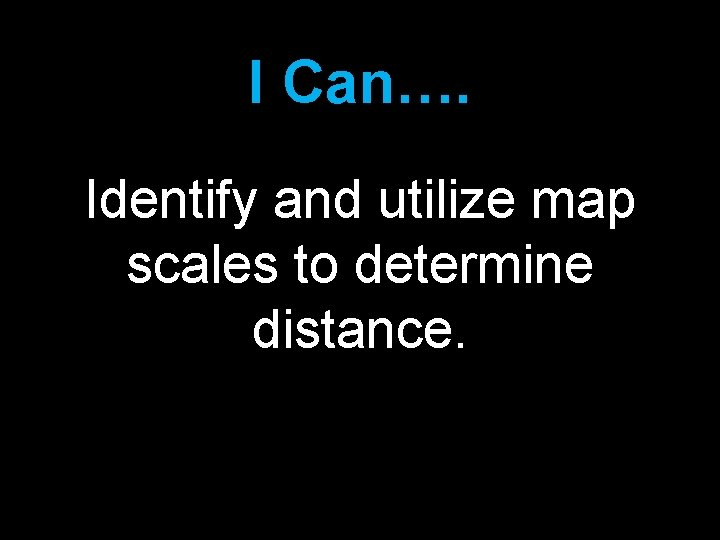I Can…. Identify and utilize map scales to determine distance.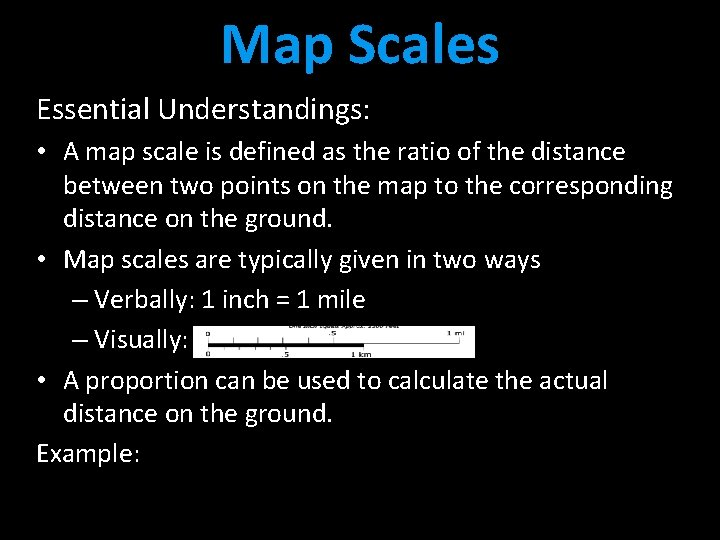Map Scales Essential Understandings: • A map scale is defined as the ratio of the distance between two points on the map to the corresponding distance on the ground. • Map scales are typically given in two ways – Verbally: 1 inch = 1 mile – Visually: • A proportion can be used to calculate the actual distance on the ground. Example: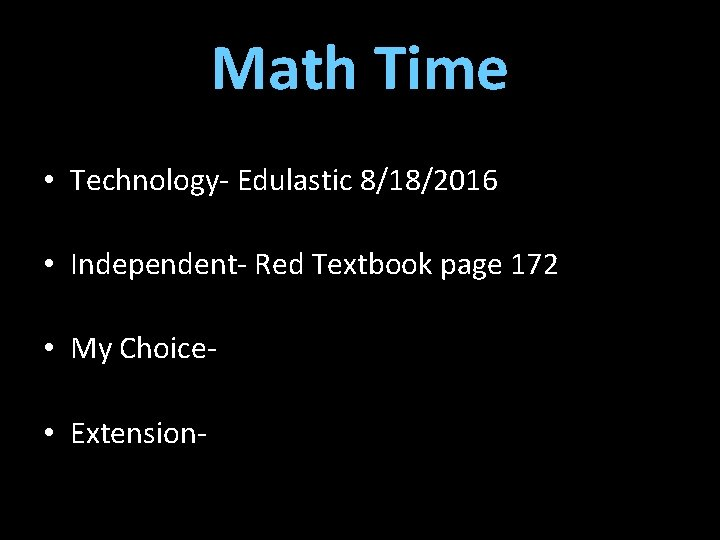Math Time • Technology- Edulastic 8/18/2016 • Independent- Red Textbook page 172 • My Choice • Extension-Wrap it Up • Review • Questions • Exit TicketsDay 5Bell Work Quietly review your vocabulary with the person who sits next to or behind you. (5 minutes)Vocabulary Equivalent- Equal or the same. Fraction- A comparison of two numbers by division. A ratio that compares a part to the whole or set. Percentage- A ratio used to compare parts per 100. Represents a part to whole relationship. Proportion- An equation composed of two equivalent ratios. Rate- A ratio used to compare quantities with different units of measure. Rate of Change- Describes how one quantity changes in relation to another quantity. Ratio- A comparison of two or more quantities. Ratio Table- A table composed of equivalent ratios. Scaling- To increase or decrease a ratio, using proportional reasoning. Unit Rate- A rate decreased to a comparison of 1.Quick Quiz Please clear your desk of everything except for a pencil and the provided quiz.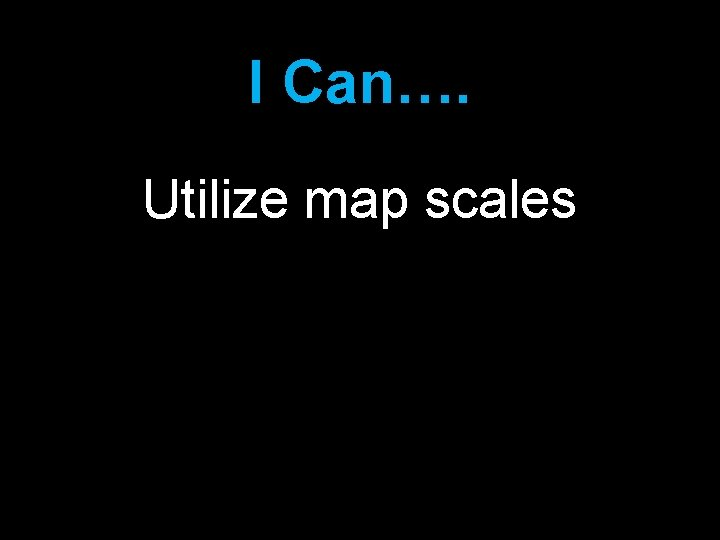I Can…. Utilize map scales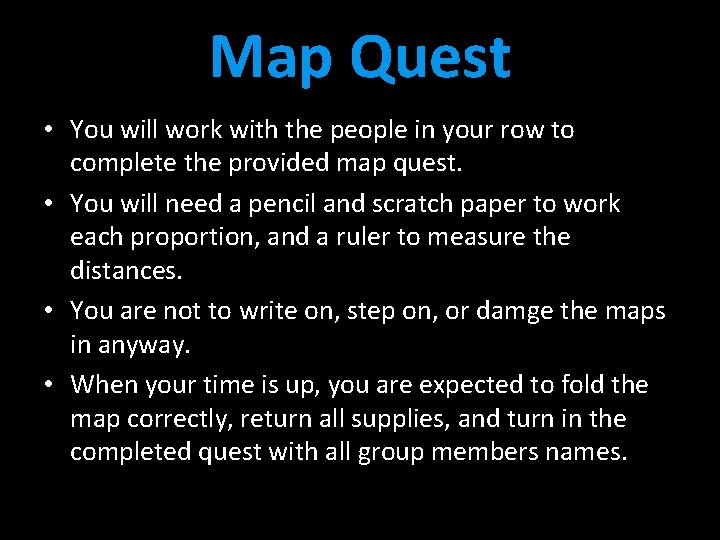Map Quest • You will work with the people in your row to complete the provided map quest. • You will need a pencil and scratch paper to work each proportion, and a ruler to measure the distances. • You are not to write on, step on, or damge the maps in anyway. • When your time is up, you are expected to fold the map correctly, return all supplies, and turn in the completed quest with all group members names.Wrap it Up • Review • Questions • Exit Tickets